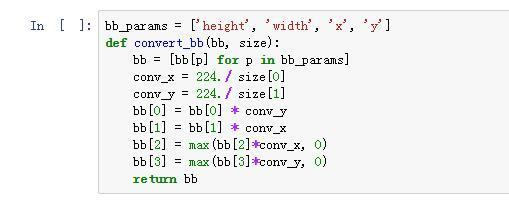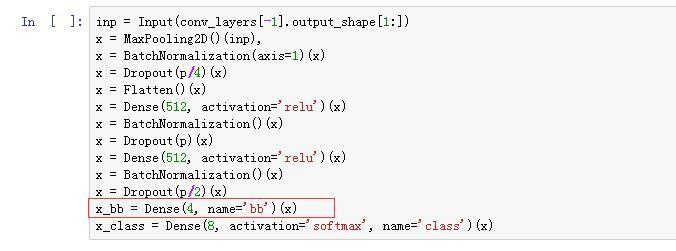# Lesson 7 discussion

I put together notes on lesson 7 with a bunch of questions inlineWould be great if you all can edit it, if you understand those aspects better: http://wiki.fast.ai/index.php/Lesson-7-notes

Hey Sravya,

The former is what I was facing initially too. My performance with Resnet even after adding shuffle=False remains worse than VGG + batch norm.

``````   batches = get_batches(path+'train',shuffle=False,batch_size=64)
val_batches = get_batches(path+'valid',shuffle=False,batch_size=64)
test_batches = get_batches(test_path,shuffle=False,batch_size=64)
``````

Any pointers to what might be going wrong? I’m clipping my predictions at 0.05 and 0.975.
Thanks!

For those of you using resnet, which layers are you making trainable? You’ll need to be careful to choose a set of layers that makes sense given the architecture of resnet!

@sravya8 note that @bckenstler has finished the lesson 7 notes now: http://wiki.fast.ai/index.php/Lesson_7_Notes

Also, here’s the data leakage paper - http://www.cs.umb.edu/~ding/history/470_670_fall_2011/papers/cs670_Tran_PreferredPaper_LeakingInDataMining.pdf . (Brad perhaps you could link to that from the notes?)

I’ve been looking at Lesson 7 and, in particular, generating the output from the convolution layers and I am confused by the fact that you have many different files for the weights.

If I look at vgg16.py, when the model is built we load the weights ‘vgg16.h5’ while in vgg16bn.py, if there is no top you we load ‘vgg16_bn_conv.h5’ and with the top we load ‘vgg16_bn.h5’. I can understand that ‘vgg16_bn.h5’ should be different from ‘vgg16.h5’ for the dense layers due to the addition of batch normalization but since the convolution layers don’t change, I would expect ‘vgg16_bn_conv.h5’ to be identical to ‘vgg16.h5’ without the top.

Also in lesson 7, to get the output of the convolution layers, you are using the VGG16BN model but before splitting off the convolution layers, the model is trained for 3 epochs and the weights are saved. These weights are reloaded just before the splitting but given that in the fine tuning process all of the layers are frozen except for the decision layer that would seem to me to be a step that would have no effect. Can you explain why you reloaded the weights?

Basically, my question is if you are only interested in getting the output of the convolution layers, whether you use the original VGG16 model or the VGG16BN model should not matter shouldn’t it? Are they not identical up until we get to the fully connected layers?

Not quite - the BN version was fine-tuned so the conv layers will have different scaling to take advantage of the BN.

Hey Chintan,

I’m facing the same issue. Did you have a chance to fix it?

Thanks!

Has anyone been experiencing the same issue that I have – in that I need to use Keras flow_from_directory() instead of flow() and fit_generator() instead of fit() – because I am using my local machine (old win7 machine with 6GB memory) and can’t load all the fisheries images into memory?

I would really like to specify numpy arrays in memory (those arrays for the metadata inputs, the bounding boxes, and the test predictions) for use with flow_from_directory() and fit_generator() data in those 2 methods. It is impacting the ability do multi-input, multi-output, and pseudo-labeling with MixIterator (the last one because the DirectoryIterator object generated by flow_from_directory does not support indexing). You cannot specify your own ‘y’ with flow_from_directory() like you can with flow() for the pseudo-labeling.

Am I missing something? Is it possible to get those 2 methods to work with arrays in memory as additional inputs to the images in the directories? Do I need to write a lot of new code to make it work? Or should I just forget it and add more memory to my machine?Thanks, Christina

1 Like

Hi all, can someone explain to me why the image size inputs were input to BatchNorm layer if it’s already normalized in notebook 7 ?

@jeremy your lessons are AMAZING. I couldn’t find a way to start a new thread, so please allow me to ask you a question here: what do I do if I want to classify something other than an image?

What really got me excited about Deep Learning from your first lesson was that you said “it’s completely false when people say deep learning requires a large data set.” I am starting to wonder if that’s only true because we have pre-trained models based on large datasets like ImageNet, and we can achieve great results by fine tuning it, if the classification problem falls under a similar category of the pre-trained model.

Let’s say I want to use deep learning to implement a speech recognition model for a very limited set of situations. From your experience, do you think I will need at least thousands of audio recordings to be able to come up with a viable model? More generally, how do I go about building a model from scratch when I can’t rely on a pre-trained model?

I am having exactly the same problem and I had to resort to a different procedure altogether without using the Mixiterator.

In the notebook, it looks like the mixiterator worked for Jeremy and he was able to fetch the precomputed batches even if they are not technically images with 3 channels. Can anyone shed any light on that?

1 Like

Can you explain tour method/ show the code ? I am stuck here and with much bigger test-stg2 dataset, pseudo labeling would help much

maclej, what I am doing is basically just taking the predictions, shuffling them alongside their respective features (the output of the conv_layers for the test set), then concatenating a number of them to the train set and shuffling again. The big downside of this is that the numpy array needs to be kept in memory. See function below:

``````def fit_predict_pl_mod(tst_preds, conv_layers, conv_trn_feat, trn_bbox_cm, \
trn_labels, conv_val_feat, val_bbox_cm, val_labels, conv_tst_feat):
(trn_len, psl_len) = (conv_trn_feat.shape, conv_trn_feat.shape / 5)
shuf_tst_ls = np.random.permutation(range(conv_tst_feat.shape))
tst_ls = [conv_tst_feat[ind,:,:,:] for ind in shuf_tst_ls][0:psl_len]
tst_lb_ls = [tst_preds[ind,:] for ind in shuf_tst_ls][0:psl_len]
all_img_ls = [conv_trn_feat[ind,:,:,:] for ind in range(conv_trn_feat.shape)] + tst_ls
all_tst_lb_ls = [trn_labels[ind,:] for ind in range(trn_labels.shape)] + tst_lb_ls
shuf_ls = np.random.permutation(range(trn_len + psl_len))
psl = np.array([all_img_ls[ind] for ind in shuf_ls])
labels = np.array([all_tst_lb_ls[ind] for ind in shuf_ls])
pl_model = create_m640(conv_layers)
pl_model.fit(psl, labels, nb_epoch=3, validation_data=(conv_val_feat, val_labels))
pl_model.optimizer.lr = 1E-5
pl_model.fit(psl, labels, nb_epoch=12, validation_data=(conv_val_feat, val_labels),
callbacks=[EarlyStopping(monitor='val_loss', patience=2)])
pl_tst_preds = pl_model.predict(conv_tst_feat, batch_size=batch_size)
return pl_tst_preds
#end
``````

Ok I get it, nice way around it, I will try it I I manage to steal time before final deadlineI would like to ask is the lesson 7 notebook test set based on test_stg1 or test_stg2 test data. ThanksI am also having a problem in that using test_stg1 data in lesson 7 cell 4 I am getting zero test images even though there are 1000 images in the test directory. Thanks

Ok fixed my problem created another directory inside test called test. Now it picks up the test images.

Any comment

Has anyone seen this error

raw_test_sizes = [PIL.Image.open(path+‘test/’+f).size for f in val_filenames]
test_sizes = to_categorical([size2id[o] for o in raw_test_sizes], len(id2size))
test_sizes = test_sizes-trn_sizes_orig.mean(axis=0)/trn_sizes_orig.std(axis=0)

.#preds = model.predict([conv_test_feat, test_sizes], batch_size=batch_size2)
preds = model.predict(conv_test_feat, batch_size=batch_size
2)

ValueError Traceback (most recent call last)
in ()
1 #preds = model.predict([conv_test_feat, test_sizes], batch_size=batch_size2)
----> 2 preds = model.predict(conv_test_feat, batch_size=batch_size
2)

/home/dl/anaconda2/lib/python2.7/site-packages/keras/engine/training.pyc in predict(self, x, batch_size, verbose)
1252 x = standardize_input_data(x, self.input_names,
1253 self.internal_input_shapes,
-> 1254 check_batch_axis=False)
1255 if self.stateful:
1256 if x.shape > batch_size and x.shape % batch_size != 0:

/home/dl/anaconda2/lib/python2.7/site-packages/keras/engine/training.pyc in standardize_input_data(data, names, shapes, check_batch_axis, exception_prefix)
122 ’ to have shape ’ + str(shapes[i]) +
123 ’ but got array with shape ’ +
–> 124 str(array.shape))
125 return arrays
126

ValueError: Error when checking : expected input_3 to have shape (None, 512, 14, 14) but got array with shape (1000, 512, 22, 40)

I pasted the top cell in line with sml0820 reply suggestion that gives different error so applied the second suggestion from sml0820 but then get the above error.

1 Like

I have 2 quentions here,
1.in this cell, why th bb and bb need ‘max()’ ? Since the coordinate wold never be negative right?2.In the bbox section, there’re 2 outputs, why the number of x_bb outputs is 4?Try printing out the arguments to the functions and have a think about what we’re trying to calculate - see if you can figure it out!If you can, let us know what you think the answer is - and if not, I can provide more info.

I got it, the dense layer outputs 4 features: height, width, x, y, it’s exactly the predict bounding box!
But I still don’t figure out the question 1…